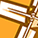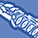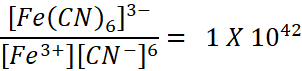cancel
Showing results for
Did you mean:New Contributor

## Cyanide Complex Formation

If I make 2 solutions:

• 7.5 x 10-4 M K3Fe(CN)6 aqueous.
• 7.5 x 10-4 M FeCl3 + 4.5 x 10-3 M KCN aqueous.

Will they both form the same (Fe(CN)6)-3 complex?  Will they both have the same lambda max?  Same absorbance?

Thank you.

Pete Williamsen

2 RepliesNew Contributor II

## Re: Cyanide Complex Formation

Spoiler

When you create two solutions, one with 7.5 x 10^-4 M K3Fe(CN)6 aqueous and the other with 7.5 x 10^-4 M FeCl3 + 4.5 x 10^-3 M KCN aqueous, both contain the components necessary to form the (Fe(CN)6)^-3 complex, but they have different starting materials.

In the first solution (K3Fe(CN)6), you already have the potassium hexacyanoferrate(III) complex dissolved in water. In the second solution (FeCl3 + KCN), you have ferric chloride (FeCl3) and potassium cyanide (KCN), which can react to form the same complex in situ.

Both solutions are expected to form the (Fe(CN)6)^-3 complex, as long as the reaction between FeCl3 and KCN proceeds as expected, converting Fe(III) into Fe(CN)6^-3.

However, the exact concentration of the complex formed might differ in the two solutions due to potential differences in reaction kinetics and conditions. Consequently, the absorbance and lambda max may not be exactly the same in both solutions. Lambda max, the wavelength at which the complex exhibits maximum absorbance, can be influenced by factors like concentration and reaction conditions.

To determine if they have the same lambda max and absorbance, you would need to measure the absorbance spectra of both solutions across a range of wavelengths and compare the resulting spectra. This experimental data would provide a more precise answer to your question.New Contributor

## Re: Cyanide Complex Formation

Thank you for your response.  I have an additional question.  The Formation Constant formula in the literature is:Is there a way to calculate the Free Cyanide, [CN-], concentration?  Assuming its the [7.5E-4] Potassium Ferricyanide solution.  I would eventually like to predict the Free Cyanide concentration based on the the iron concentration.

Thank you